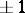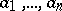# Unimodular transformation

A linear transformation of a finite-dimensional vector space whose matrix has determinant.
The name "unimodular transformation" is often restricted to mean a linear transformation with determinant. In the context of a vector spaceover a fieldwhich is the quotient field of an integral domain, with a fixed-basisin, a linear transformation is called unimodular if its matrix with respect tohas entries inand determinant a unit in. Under each of these definitions the unimodular transformations form a group. In the case of linear transformations with determinantone often calls this the unimodular group, or, more commonly nowadays, the special linear group.